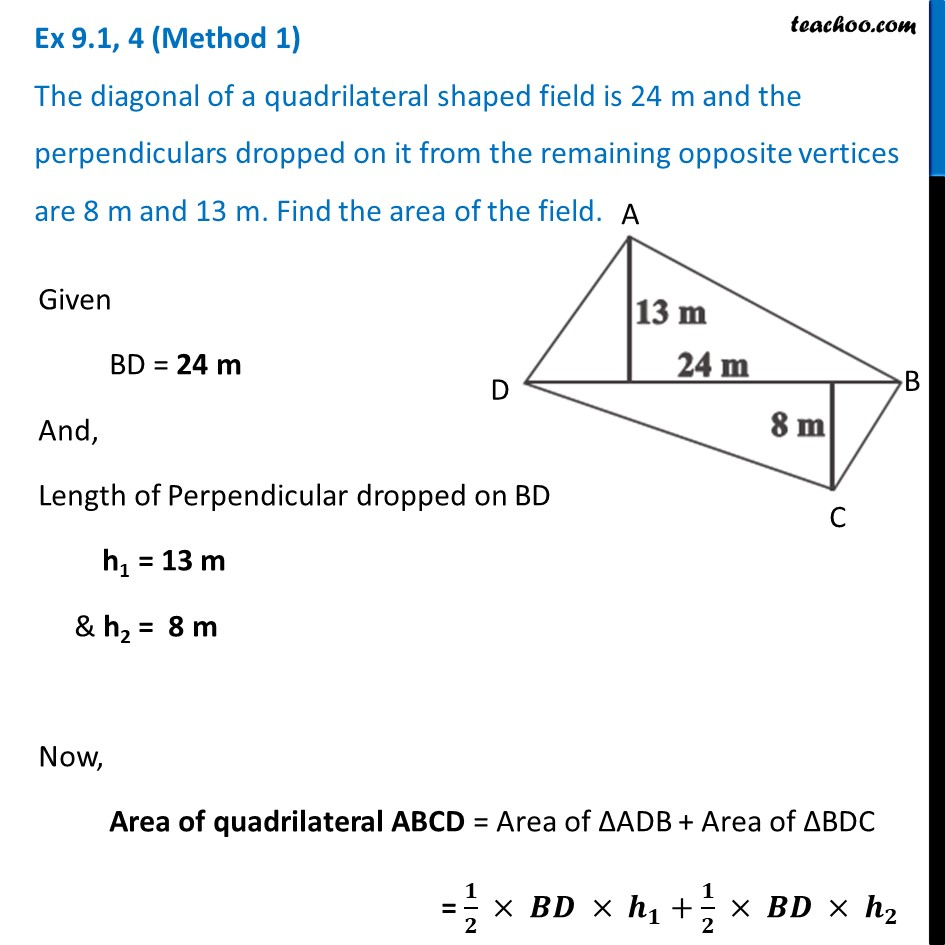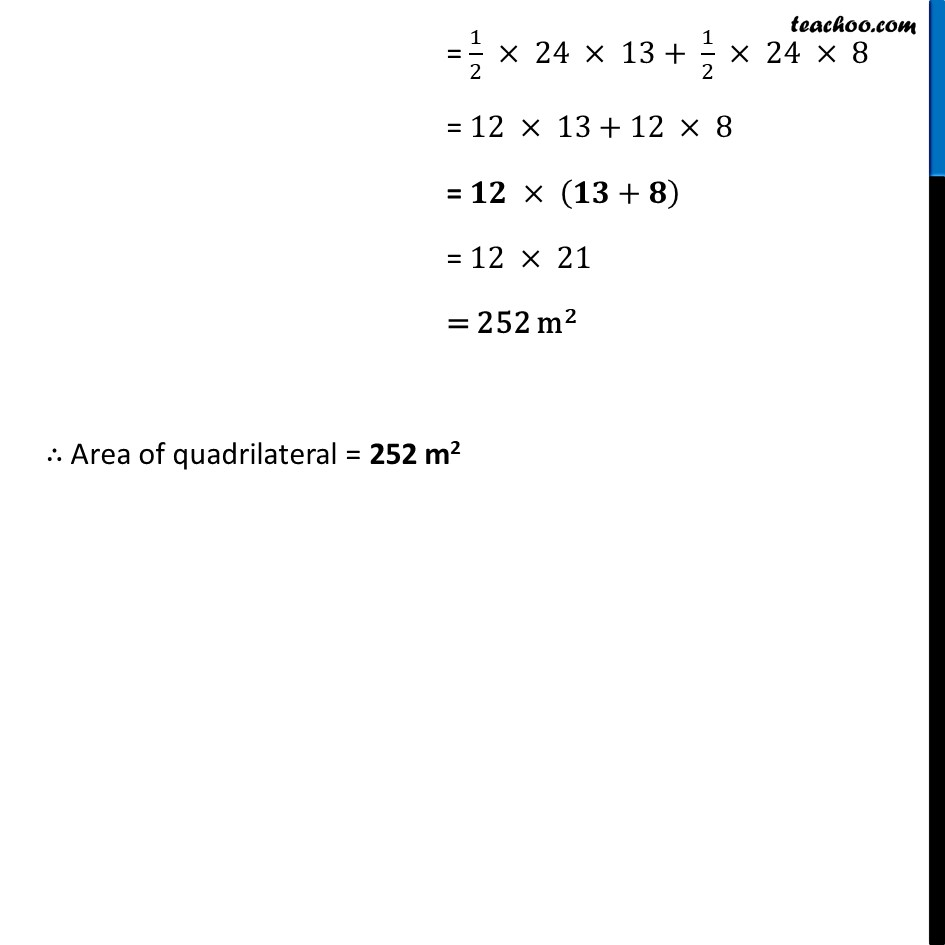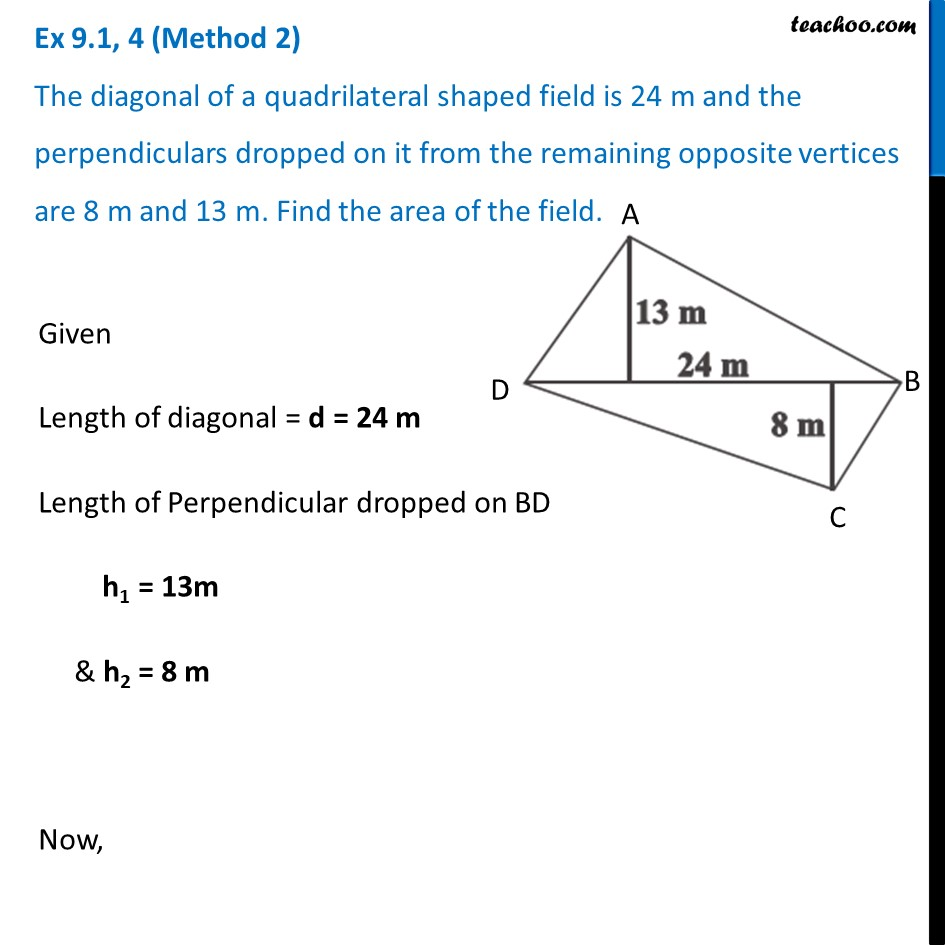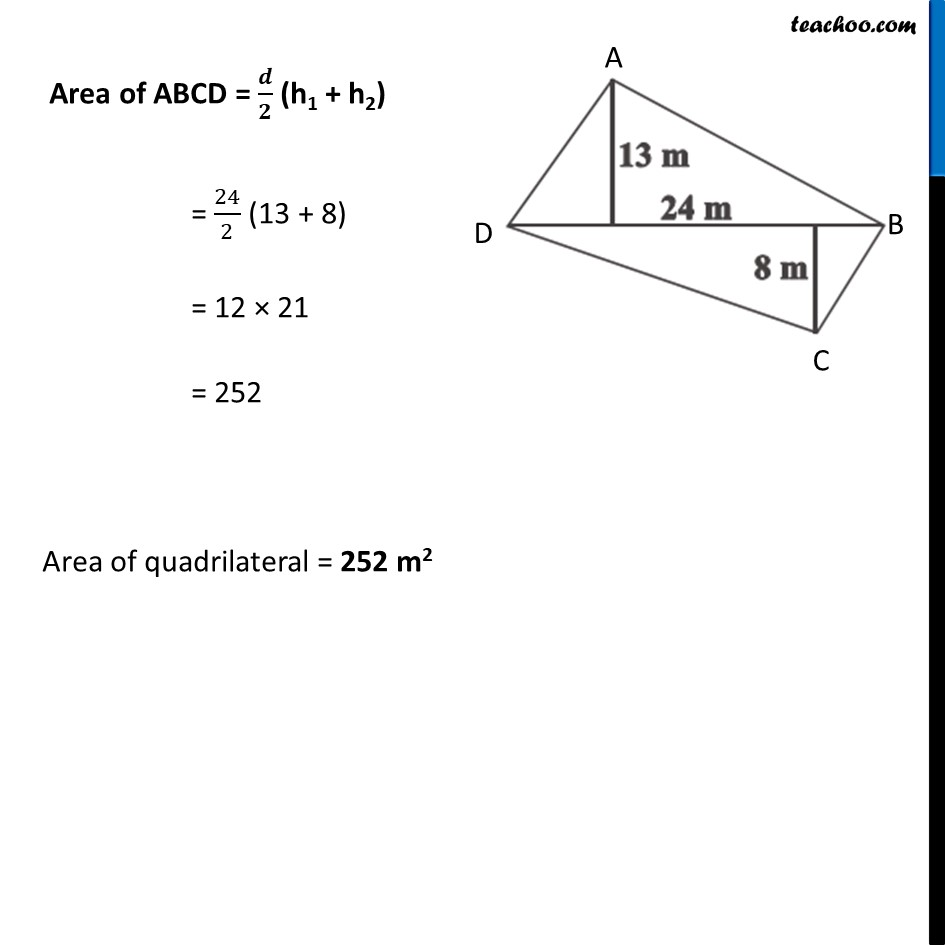Ex 9.1

Chapter 9 Class 8 Mensuration
Serial order wiseLearn in your speed, with individual attention - Teachoo Maths 1-on-1 Class

### Transcript

Ex 9.1, 4 (Method 1) The diagonal of a quadrilateral shaped field is 24 m and the perpendiculars dropped on it from the remaining opposite vertices are 8 m and 13 m. Find the area of the field.Given BD = 24 m And, Length of Perpendicular dropped on BD h1 = 13 m & h2 = 8 m Now, Area of quadrilateral ABCD = Area of ΔADB + Area of ΔBDC = 𝟏/𝟐 × 𝑩𝑫 × 𝒉_𝟏+𝟏/𝟐 × 𝑩𝑫 × 𝒉_𝟐 = 1/2 × 24 × 13+ 1/2 × 24 × 8 = 12 × 13+12 × 8 = 𝟏𝟐 × (𝟏𝟑+𝟖) = 12 × 21 = 252 m2 ∴ Area of quadrilateral = 252 m2 Ex 9.1, 4 (Method 2) The diagonal of a quadrilateral shaped field is 24 m and the perpendiculars dropped on it from the remaining opposite vertices are 8 m and 13 m. Find the area of the field.Given Length of diagonal = d = 24 m Length of Perpendicular dropped on BD h1 = 13m & h2 = 8 m Now, Area of ABCD = 𝒅/𝟐 (h1 + h2) = 24/2 (13 + 8) = 12 × 21 = 252 Area of quadrilateral = 252 m2